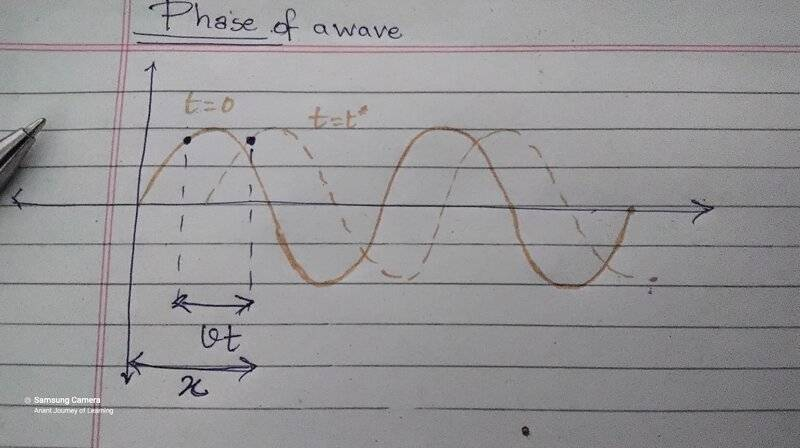# Phase of a wave - My interpretation

Shreya
Homework Statement:
I have always wondered what the phase of a wave means. I came up with something and I would really appreciate if someone could help me verify it and possibly find out flaws in it.
Relevant Equations:
My textbook says: $$y = A \sin (kx - \omega t)$$ $$y = A \sin k (x - vt)$$ $$y=A \sin (\frac {2\pi} {\lambda} (x - vt)$$ as ##\frac w k = v##
Let's begin my interpretation: (please refer the image below). There I have considered a point in the disturbance/wave (let's call it ##P##),(not a particle of the medium) and I follow it as the wave progresses. The solid curve is a Pic of the wave at ##t=0## and the dotted one is its Pic at some later time. The point in the disturbance has moved by ##vt##. So, the ##x - vt## in the equation can be interpreted as the intital distance of point P. On Dividing that by ##\lambda##, we get 'what part of the cycle was the point at initially' or the initial state. And on multiplying it by ##2\pi##, we are coverting that to 'what part of a complete revolution'. Finally taking the ##\sin## of it and multiplying with ##A## we get the ##y## value.

I would love to see comments on this & Understand any mistakes I've made. Thanks in Advance.
(Edit: Sorry for the blurry image, I have updated it)Last edited:

Homework Helper
Gold Member
The definition of phase is the argument of the harmonic function, i.e. what's between the parentheses. It the angle the sine (or cosine) of which you are to consider. In a traveling wave a constant phase travels at the speed of propagation of the wave.

•Shreya
Shreya
The definition of phase is the argument of the harmonic function, i.e. what's between the parentheses. It the angle the sine (or cosine) of which you are to consider
Yes, I understand that. But, my attempt above was to justify why it is what it is or, rather, why we put ##kx-\omega t## as the phase.

Last edited:
Homework Helper
Gold Member
Yes, I understand that. But, my attempt above was to justify why it is what it is or, rather, why we put ##kx-\omega t## as the phase.
Because that is how we describe a wave traveling to the right. If you look at the equivalent formulation ##y=A \sin \large(\frac {2\pi} {\lambda} (x - vt)\large)##, you will clearly see why that is. As ##t## increases (which it always does) ##x## must also increase to keep the phase constant. In other words the constant phase travels at speed ##v##.

Look at the square dot in your drawing. Assume that at ##t=0## its coordinates are ##(x_0,y_0)##. At ##t=t_1## you want ##y(x_1,t_1) = y(x_0,0)## units. For ##y## to have the same value at the two different times and positions, the phase must be the same, $$\frac {2\pi} {\lambda} (x_1 - vt_1)=\frac {2\pi} {\lambda} (x_0 - 0) \implies x_1-x_0=vt_1$$This says that the square dot is at distance ##vt_1## away from where it started. In other words, the constant phase travels at speed ##v##.

You can consolidate your understanding and use similar reasoning to convince yourself that ##y=A \sin \large(\frac {2\pi} {\lambda} (x + vt)\large)## describes a wave traveling to the left.

On edit: Deleted extraneous ##=0## in the equation.

Last edited:
•Shreya
Shreya
Because that is how we describe a wave traveling to the right. If you look at the equivalent formulation ##y=A \sin \large(\frac {2\pi} {\lambda} (x - vt)\large)##, you will clearly see why that is. As ##t## increases (which it always does) ##x## must also increase to keep the phase constant. In other words the constant phase travels at speed ##v##.

Look at the square dot in your drawing. Assume that at ##t=0## its coordinates are ##(x_0,y_0)##. At ##t=t_1## you want ##y(x_1,t_1) = y(x_0,0)## units. For ##y## to have the same value at the two different times and positions, the phase must be the same, $$\frac {2\pi} {\lambda} (x_1 - vt_1)=\frac {2\pi} {\lambda} (x_0 - 0)=0 \implies x_1-x_0=vt_1$$This says that the square dot is at distance ##vt_1## away from where it started. In other words, the constant phase travels at speed ##v##.

You can consolidate your understanding and use similar reasoning to convince yourself that ##y=A \sin \large(\frac {2\pi} {\lambda} (x + vt)\large)## describes a wave traveling to the left.
Thanks a million @kuruman, I had this question in the back of my mind for months. Now I can see why the phase is so, and I can also understand what it means for phase to be constant . 🙏☺

Ps: Sorry for the late reply. I'm from a different time zone.

•kuruman